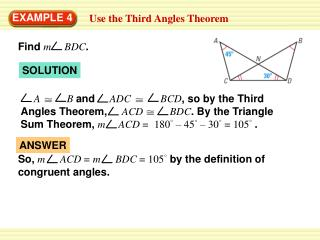Download PresentationEXAMPLE 4

# EXAMPLE 4 - PowerPoint PPT PresentationDownload Presentation## EXAMPLE 4

- - - - - - - - - - - - - - - - - - - - - - - - - - - E N D - - - - - - - - - - - - - - - - - - - - - - - - - - -
##### Presentation Transcript

1. FindmBDC. SOLUTION AB and ADCBCD, so by the Third Angles Theorem, ACDBDC. By the Triangle Sum Theorem, m ACD = 180°– 45° – 30°= 105°. So, mACD =mBDC = 105° by the definition of congruent angles. ANSWER EXAMPLE 4 Use the Third Angles Theorem

2. Write a proof. GIVEN AD CB,DC AB CADACB ACDCAB, ACDCAB PROVE Use the Reflexive Property to show that AC AC. Use the Third Angles Theorem to show that BD EXAMPLE 5 Prove that triangles are congruent Plan for Proof

3. STATEMENTS REASONS ,DC BA AD CB Given Reflexive Property of Congruence AC AC. ACDCAB, Given CADACB BD Third Angles Theorem ACDCAB Definition of EXAMPLE 5 Prove that triangles are congruent Plan in Action

4. In the diagram, what is m DCN. CDN NSR, DNC SNRthen the third angles are also congruent NRS DCN = 75° for Examples 4 and 5 GUIDED PRACTICE SOLUTION

5. By the definition of congruence, what additional information is needed to know that NDC NSR. ANSWER DC RS and DN SN for Examples 4 and 5 GUIDED PRACTICE